Diagram geometry

A diagram is like a blueprint describing a possible combination of-dimensional incidence structures in a higher-dimensional geometry (cf. Incidence system). In this context, a geometry of rankis a connected graphequipped with an-partitionsuch that every maximal clique ofmeets every class of. The vertices and the adjacency relation ofare the elements and the incidence relation of the geometry, the cliques ofare called flags, the neighbourhood of a non-maximal flag is its residue, the classes ofare called types and the type (respectively, rank) of a residue is the set (respectively, number) of types met by it. Given a catalogue of "nice" geometries of rankand a symbol for each of those classes, one can compose diagrams of any rank by those symbols. The nodes of a diagram represent types and, by attaching a label to the stroke connecting two typesandor by some other convention, one indicates which class the residues of type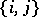should belong to. For instance, a simple strokea double strokeand the "null stroke"are normally used to denote, respectively, the class of projective planes (cf. Projective plane), the class of generalized quadrangles [a5] (cf. also Quadrangle) and the class of generalized digons (generalized digons are the trivial geometry of rank, where any two elements of different type are incident). The following two diagrams are drawn according to these conventions.

1)2)They both represent geometries of rank, where the residues of type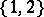are projective planes and the residues of typeare generalized digons. However, the residues of type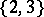are projective planes in 1) and generalized quadrangles in 2). The following is a typical problem in diagram geometry: given a diagram of such-and-such type, classify the geometries that fit with it, or at least the finite or flag-transitive such geometries (a geometryis said to be flag-transitive if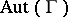acts transitively on the set of maximal flags of). In the "best" cases the answer is as follows: the geometries under consideration belong to a certain well-known family or, possibly, arise in connection with certain small or sporadic groups. For instance, it is not difficult to prove that-dimensional projective geometries are the only examples for the above diagram 1). On the other hand, the following has been proved quite recently [a7]: Ifis a flag-transitive example for the diagram 2) and if all rank-residues ofare finite of size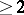, thenis either a classical polar space [a6] (arising from a non-degenerate alternating, quadratic or Hermitian form of Witt index) or a uniquely determined very small geometry with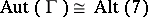.

See [a3] for a survey of related theorems.